# Orthogonal matrix

(diff) ← Older revision | Latest revision (diff) | Newer revision → (diff)

A matrix over a commutative ringwith identityfor which the transposed matrix coincides with the inverse. The determinant of an orthogonal matrix is equal to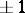. The set of all orthogonal matrices of orderoverforms a subgroup of the general linear group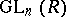. For any real orthogonal matrixthere is a real orthogonal matrixsuch that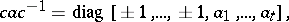where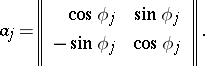A non-singular complex matrixis similar to a complex orthogonal matrix if and only if its system of elementary divisors possesses the following properties:

1) for, the elementary divisorsand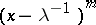are repeated the same number of times;

2) each elementary divisor of the form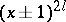is repeated an even number of times.

How to Cite This Entry:
Orthogonal matrix. Encyclopedia of Mathematics. URL: http://encyclopediaofmath.org/index.php?title=Orthogonal_matrix&oldid=17418
This article was adapted from an original article by D.A. Suprunenko (originator), which appeared in Encyclopedia of Mathematics - ISBN 1402006098. See original article